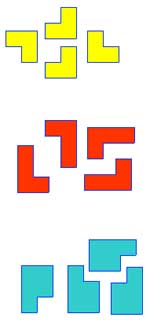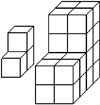Make enlargements of the coloured shapes by fitting together four identical smaller shapes.

How much longer are the edges (the linear scale factor of the enlargement)?

How much bigger is the area (the area scale factor of the enlargement)?

Now look at the illustration of the 3-dimensional solids. One is an enlargement of the other.What is the linear scale factor of the enlargement?

How much bigger is the surface area (the area scale factor)?

How much bigger is the volume (the volume scale factor)?